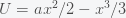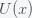# The Probability of Life

Posted on the 06 April 2019 by Ccc1685 @ccc1685

Estimates from the Kepler satellite suggest that there could be at least 40 billion exoplanets capable of supporting life in our galaxy alone. Given that there are perhaps 2 trillion observable galaxies, that amounts to a lot of places where life could exist. And this is only counting biochemical life as we know it. There could also be non-biochemical lifeforms that we can't even imagine. I chatted with mathematician Morris Hirsch a long ago in Berkeley and he whimsically suggested that there could be creatures living in astrophysical plasmas, which are highly nonlinear. So let's be generous and say that there areplanets for biochemical life to exist in the milky way andtotal in the observable universe.

Now, does this mean that extraterrestrial life is very likely? If you listen to most astronomers and astrobiologists these days, it would seem that life is guaranteed to be out there and we just need to build a big enough telescope to find it. There are several missions in the works to detect signatures of life like methane or oxygen in the atmosphere of an exoplanet. However, the likelihood of life outside of the earth is predicated on the probability of forming life anywhere and we have no idea what that number is. Although it only took about a billion years for life to form on earth that does not really gives us any information for how likely it will form elsewhere.

Here is a simple example to illustrate how life could grow exponentially fast after it forms but take an arbitrarily long time to form. Suppose the biomass of life on a planet,, obeys the simple equationwhereis a zero mean stochastic forcing with variance. The deterministic equation has two fixed points, a stable one atand an unstable one at. Thus as long asis smaller thanlife will never form but as soon asexceedsit will grow (super-exponentially), which will then be damped by nonlinear processes that I don't consider. We can rewrite this problem aswhere.The probability of life is then given by the probability of escape from the wellgiven noisy forcing (thermal bath) provided by. By Kramer's escape rate (which you can look up or I'll derive in a future post), the rate of escape is given approximately by, whereis the well depth, which is given by. Thus the probability of life is exponentially damped by a factor of. Given that we know nothing aboutorthe probability of life could be anything. For example, if we arbitrarily assignand, we get a rate (or probability if we normalize correctly) for life to be on the order of, which is very small indeed and makes life very unlikely in the universe.

Now, how could it be that there is any life in the universe at all if it had such a low probability to form at all. Well, there is no reason that there could have been lots of universes, which is what string theory and cosmology now predict. Maybe it tookuniverses to exist before life formed. I'm not saying that there is no one out there, I'm only saying that an N of one does not give us much information about how likely that is.

See more